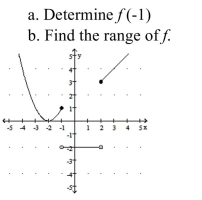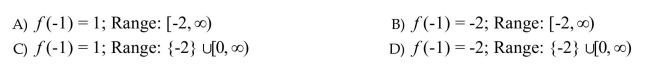# [Solved] Use the Graph of Y = F (X) to Answer

Question 140
Multiple Choice

## Use the graph of y = f (x) to answer the questions. -10+ million students use Quizplus to study and prepare for their homework, quizzes and exams through 20m+ questions in 300k quizzes.

### Mathematics

Explore our library and get Calculus Homework Help with various study sets and a huge amount of quizzes and questions

133

Study sets

2K

Quizzes

228.8K

Questions

Upload material to get free accessInvite a friend and get free accessSubscribe and get an instant access